## ML Aggarwal Class 7 Solutions for ICSE Maths Chapter 7 Percentage and Its applications Ex 7.1

Question 1.
Convert the following percents into fractions in simplest form:
(i) 25%
(ii) 150%
(iii) 7$$\frac { 1 }{ 2 }$$ %
(iv) 33$$\frac { 1 }{ 3 }$$ %
Solution: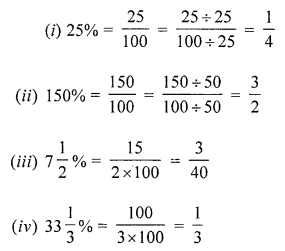Question 2.
Convert the following fractions into percents:
(i) $$\frac { 1 }{ 8 }$$
(ii) $$\frac { 5 }{ 4 }$$
(iii) $$\frac { 9 }{ 16 }$$
(iv) $$\frac { 3 }{ 7 }$$
(v) $$\frac { 11 }{ 15 }$$
(vi) 1$$\frac { 3 }{ 8 }$$
Solution: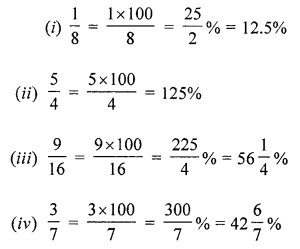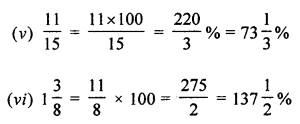Question 3.
(i) 6 students out of 40 students in a class are absent. What percentage of students are absent ?
(ii) Antony secured 384 marks out of 500 marks. Find the percentage of marks secured by Antony.
(iii) A shop has 500 shirts, out of which 15 are defective. What percentage of shirts are defective?
(iv) Vani has a collection of bangles. She has 20 gold bangles and 10 silver bangles. What is the percentage of each type of bangles?
(v) There are 120 voters and 90 of them voted. What percent did not vote?
Solution:
(i) 6 students out of 40 are absent
$$\frac { 6 }{ 40 }$$ × 100 = $$\frac { 600 }{ 40 }$$ = 15%
(ii) Antony secured 384 marks out of 500 marks
Percentage of secured marks = $$\frac { 384 }{ 500 }$$ × 100 = 76$$\frac { 1 }{ 5 }$$ % = 76.8%
(iii) A shop has 500 shirts
Defective shirts = 15
Percentage of defective shirts = $$\frac { 15 }{ 500 }$$ × 100 = 3%
(iv) Vani has 20 gold and 10 silver bangles
Total bangles = 30
Percentage of gold bangles = $$\frac { 20 }{ 30 }$$ × 100 = $$\frac { 200 }{ 3 }$$ = 66$$\frac { 2 }{ 3 }$$ %
Percentage of silver bangles = $$\frac { 10 }{ 30 }$$ × 100 = 33$$\frac { 1 }{ 3 }$$ %
(v) Out of 120 votes, 90 of them voted
Those who does not voted = 120 – 90 = 30
Percentage = $$\frac { 30 }{ 120 }$$ × 100 = 25%

Question 4.
Estimate the part of the figure which is shaded and hence find the percentage of the part which is shaded.Solution: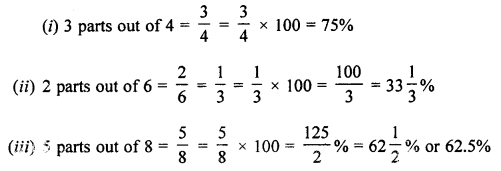Question 5.
Convert the following percentage into ratios in sirup rest form:
(i) 14 %
(ii) 1$$\frac { 3 }{ 4 }$$ %
(iii) 33$$\frac { 1 }{ 3 }$$ %
(iv) 37.5 %
Solution: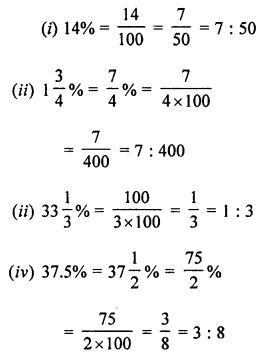Question 6.
Express the following ratios as percentages:
(i) 5 : 4
(ii) 1 : 1
(iii) 2 : 3
(iv) 9 : 16
Solution:Question 7.
An alloy consists of 7 parts of zinc and 33 parts of copper. Find the percentage of copper in the alloy ?
Solution:
In an alloy,
7 parts of zinc and 33 parts of copper
Total parts of alloy = 7 + 33 = 40 parts
33 parts out of 40 parts is copper
Percentage of copper = $$\frac { 33 }{ 40 }$$ × 100
= $$\frac { 165 }{ 2 }$$ %
= 82.5%

Question 8.
Chalk contains calcium, carbon and sand in the ratio 12 : 3 : 10. Find the percentage of carbon in the chalk 12%.
Solution:
In chalk, ratio of calcium, carbon and sand = 12 : 3 : 10.
Total of ratio’s =12 + 3 + 10 = 25 and carbon = 3
Percentage of carbon in the chalk 3
= $$\frac { 3 }{ 25 }$$ × 100
= 12%

Question 9.
If ₹ 2500 is to be divided among Ravi, Raju and Roy, so that Ravi gets two parts, Raju three parts and Roy five parts. How much money will each get? What will it be in percentages?
Solution:
Total amount = ₹ 2500
Ravi gets = 2 parts
Raju gets = 3 parts
and Roy gets = 5 parts
Total parts = 10
Ravi will get = $$\frac { 2 }{ 10 }$$ × 2500 = ₹ 500
= $$\frac { 2 }{ 10 }$$ × 100 = 20%
Raju will get = $$\frac { 3 }{ 10 }$$ × 2500 = ₹ 750
= $$\frac { 3 }{ 10 }$$ × 100 = 30%
Roy will get = $$\frac { 5 }{ 10 }$$ × 2500 = ₹ 1250
= $$\frac { 5 }{ 10 }$$ × 100 = 50%

Question 10.
Convert the following percentages to decimals:
(i) 28%
(ii) 3%
(iii) 0. 44%
(iv) 37$$\frac { 1 }{ 2 }$$ %
Solution:Question 11.
Convert the following decimals to percents:
(i) 0.65
(ii) 0.9
(iii) 2.1
(iv) 0.02
Solution: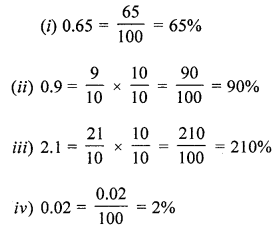Question 12.
(i) If 42% of the students in a class are girls, then what percent of the students in the class are boys?
(ii) We have a basket full of apples, oranges, and mangoes if 50% are apples, 30% are oranges, then what percent are mangoes?
Solution
(i) Number of girls in a class = 42%
Number of boys = 100 – 42 = 58%
(ii) Apples = 50%
Oranges = 30%
and rest mangoes = [100- (50 + 30)]% = (100 – 80)% = 20%